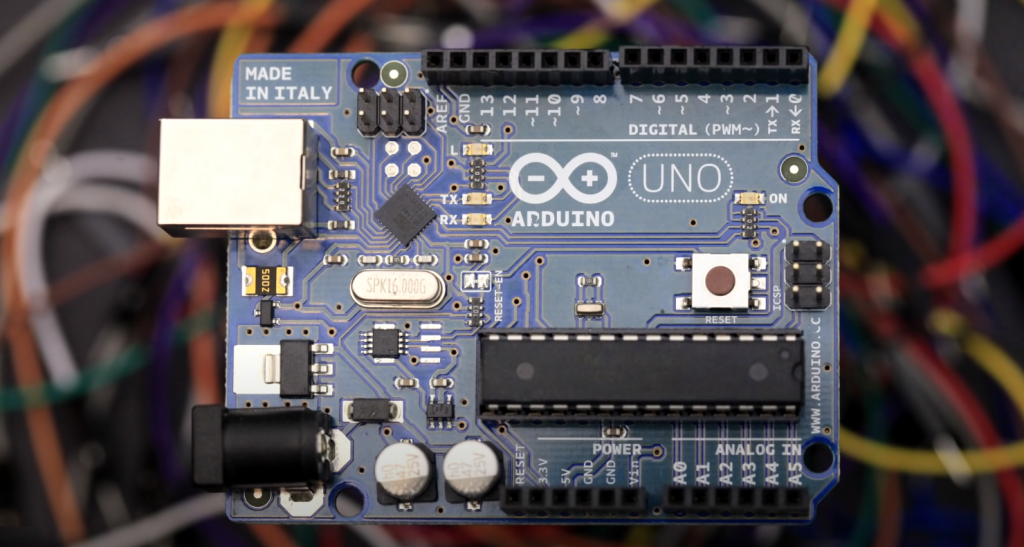# Converting Arduino char to int Guide for Beginners

Converting int integer variables to char variables in the Arduino IDE is a fairly simple process but worthy of a separate reminder. The hardest thing to understand is why the Char type is needed at all. Let me remind you that it is a one-byte character type.

To convert Int into Char, you must first convert an integer into String and then convert String into an array of Char. It’s so twisted on purpose that no one would guess.

Let’s start with the more straightforward option of converting a Char character to an Integer and then move on to the fundamental question.

Contents

## Step 1: Char to Integer

Use the following short combination to convert char to integer:

```int a;
char b;
a=b-'0';
```

And that’s it!

## Step 2: Integer to Char

The next example will use the auxiliary variable String. And the hardest part here is the conversion to an array of characters using a special function.

The code looks like this:

```int a=1;
char b;
String str;
str=String(a);
str.toCharArray(b,2);
```

Using this example, you will convert an integer to a char. However, the resulting array will only hold numbers between -9 and 99. For more digits, you would need to increase the size of the array, and instead of writing.

```char b;
```

You will have to insert

```char b;
```

That way, you can display an integer that takes up five characters. You can also use an array of char and other lengths.

## Final Words

If you are a beginner at using Arduino, then this guide was written for you. It is not difficult to convert a char to an int, and this guide walks you through each step so that you can successfully complete the task.

Thank you for your attention! See you soon!

Scroll to Top